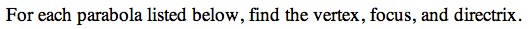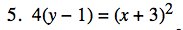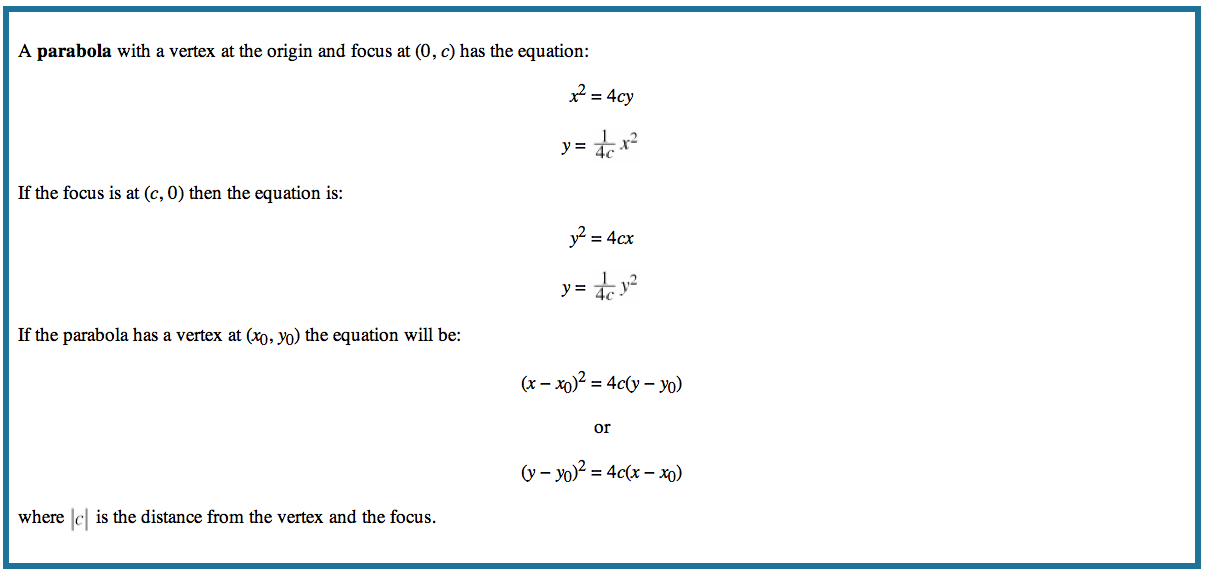13P-5.

### Missing problem for Book PCT => Chapter Ch13 => Lesson 13.1.4 => Problem 13P-5

Created from orphaned homework help problemWhat is the vertical and horizontal shift of the equation? How does this affect the vertex?